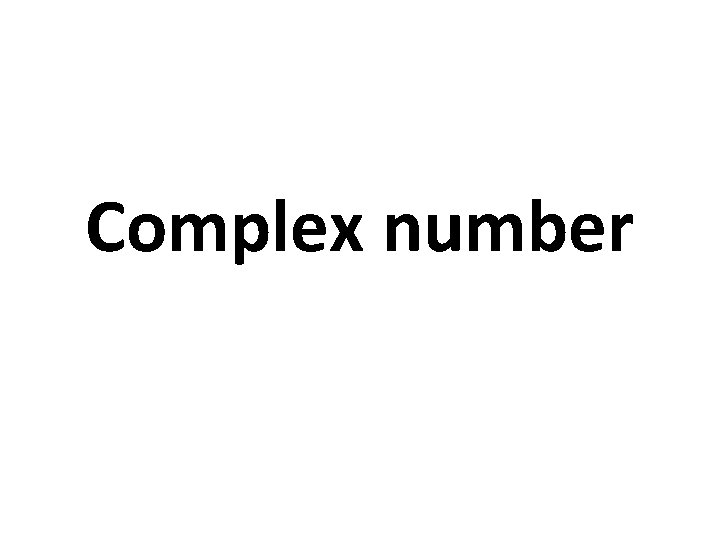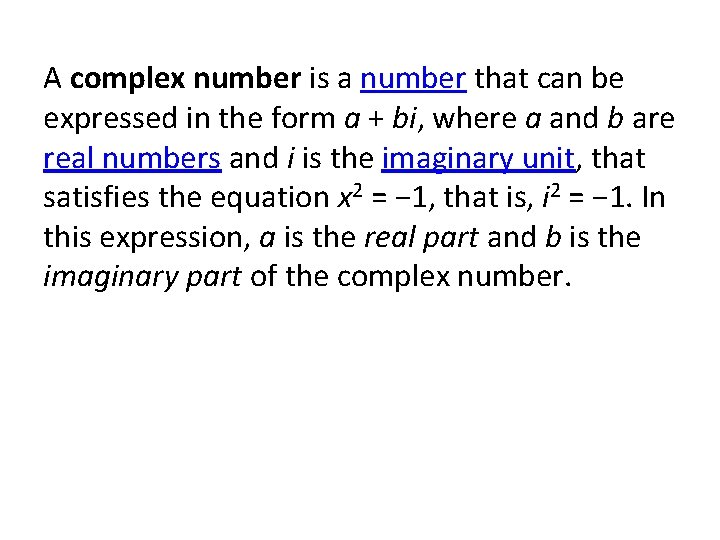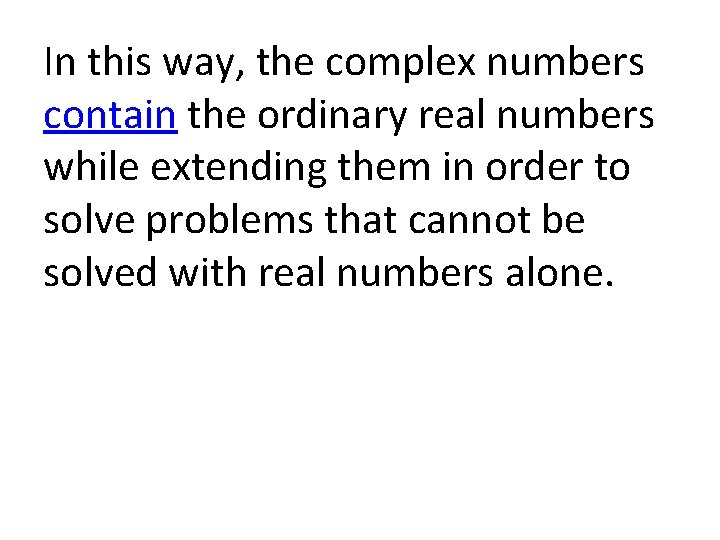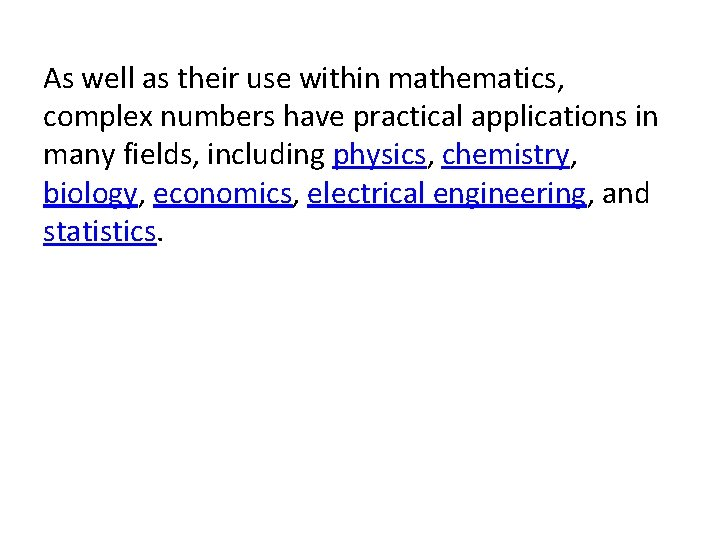# Complex number A complex number is a number

• Slides: 9Complex numberA complex number is a number that can be expressed in the form a + bi, where a and b are real numbers and i is the imaginary unit, that satisfies the equation x 2 = − 1, that is, i 2 = − 1. In this expression, a is the real part and b is the imaginary part of the complex number.Complex numbers extend the concept of the one-dimensional number line to the twodimensional complex plane (also called Argand plane) by using the horizontal axis for the real part and the vertical axis for the imaginary part.The complex number a + bi can be identified with the point (a, b) in the complex plane.A complex number whose real part is zero is said to be purely imaginary, whereas a complex number whose imaginary part is zero is a real number.In this way, the complex numbers contain the ordinary real numbers while extending them in order to solve problems that cannot be solved with real numbers alone.As well as their use within mathematics, complex numbers have practical applications in many fields, including physics, chemistry, biology, economics, electrical engineering, and statistics.The Italian mathematician Gerolamo Cardano is the first known to have introduced complex numbers.He called them "fictitious" during his attempts to find solutions to cubic equations in the 16 th century.